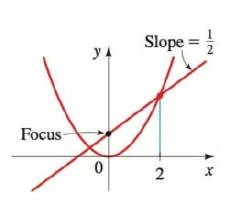Chapter 12.1, Problem 58E### Algebra and Trigonometry (MindTap ...

4th Edition
James Stewart + 2 others
ISBN: 9781305071742

#### Solutions

Chapter
Section### Algebra and Trigonometry (MindTap ...

4th Edition
James Stewart + 2 others
ISBN: 9781305071742
Textbook Problem

# 49-58 ■ Finding the Equation of a Parabola Find an equation of the parabola whose graph is shown.To determine

To find:

The equation for the parabola of given figure.

Explanation

Given:

The equation of the parabola whose graph is shown below

Approach:

The general equation for a parabola with a vertical axis having the vertex is

x2=4py …… (1)

Here, p is the distance from the origin and the vertex is V(0,0).

The focus is F(0,p) and the directrix line is y=p

The parabola opens to the upward if p>0 or to the downward if p<0.

Calculation:

From the given figure,

The shaded region has an area is 8.

So, the area of the half of the shaded region is 4

The area of the half of the shaded region from given figure and equate it to 4.

### Still sussing out bartleby?

Check out a sample textbook solution.

See a sample solution

#### The Solution to Your Study Problems

Bartleby provides explanations to thousands of textbook problems written by our experts, many with advanced degrees!

Get Started